# A Malicious Webpage Detection Algorithm Based on Image Semantics

A Malicious Webpage Detection Algorithm Based on Image Semantics
School of Software, Nanchang University, Nanchang 330047, China

Department of Computer Science and Technology, Nanchang University, Nanchang 330031, China

School of Software Engineering, East China Normal University, Shanghai 200241, China

Corresponding Author Email:
8003117137@email.ncu.edu.cn
Page:
113-118
|
DOI:
https://doi.org/10.18280/ts.370115
15 July 2019
|
Accepted:
20 November 2019
|
Published:
29 February 2020
| Citation

OPEN ACCESS

Abstract:

In the era of the Internet, malicious attacks have put user information at risk. Many malicious webpages use images as the carrier of malicious codes. If extracted accurately, the features of these images will help to improve the detection of malicious webpages. This paper aims to develop an accurate malicious webpage detection method based on the features of the said images.  Since static images contain a small amount of information, semantic segmentation was performed to predict the semantics of the target attitude. Then, the final semantics of target the image were derived by the backpropagation neural network (BPNN). After that, the image semantics were fused with the other features of the malicious webpage, and sent to the classifier for recognition. Finally, the proposed algorithm was tested on an actual dataset, in comparison with other malicious webpage detection methods. The results show that our algorithm can accurately detect malicious webpages, thanks to the introduction of image semantic features.

Keywords:

deep learning, malicious attack, image semantics, backpropagation neural network (BPNN)

1. Introduction

With the proliferation of the Internet, malicious attacks have put user information at risk. For example, Internet users might suffer from information leak after entering a phishing webpage. The leaked information could be highly sensitive, such as passwords and credit card number.

The malicious code of the phishing webpage cannot replicate on its own. To spread the code across the Internet, malicious attacks are often launched in three ways: sending a junk email with clickbait tile and keywords; creating a fake e-payment scenario; displaying texts and images embedded with links to malicious webpage. Once a user opens the email, completes the payment or clicks on the texts/images, his/her private information will be stolen and his/her computer will be infected with Trojan viruses.

To keep user information safe, it is necessary to identify the malicious webpages and prevent users from clicking on them. Considering their sheer number, the malicious webpages should be detected with the aid of machine intelligence. During the detection, special attention should be paid to the texts on each webpage, which are the main content on traditional webpages and the focal point of user-webpage interaction. The images, an emerging type of information carrier, should also be considered in the detection of malicious webpages.

Many malicious webpages have similar structures and visual features as the target webpages of Internet users. Hence, a possible way to identify malicious webpages is to evaluate the visual similarity between webpages. This calls for effective extraction of image features from each webpage.

This paper attempts to develop an accurate method for malicious webpage detection based on the image features on such webpages. Firstly, Mask region-convolutional neural network (Mask R-CNN) was improved to extract image features. Then, the complex semantics of the target image were predicted, using Kinect-based action matching and backpropagation neural network (BPNN). After that, the features of the target webpage were synthetized, and sent to the classifier for recognition. The accuracy of our algorithm was verified through contrastive experiments.

2. Literature Review

Traditionally, malicious webpages are identified by comparing each webpage against black and white list (BWL), statistical weighting and similarity judgment. For BWL comparison, the features of uniform resource locator (URL) are obtained through machine learning, and used to build a BWL database for contrastive analysis. In statistical weighting, the phishing webpages are detected by computing the term frequency–inverse document frequency (TF-IDF) of the keywords in the texts, because most malicious websites are about gambling and pornography. In similarity judgement, the phishing webpages are evaluated against normal webpages in page structure and logo image .

Based on machine learning, heuristic engines can recognize unknown webpages by training the page features. Using feedback supper vector machine (SVM), Barraclough et al.  classified and identified phishing websites through incremental sample test. Hans et al.  analyzed the main features of phishing websites, and relied on random forest and reinforcement learning to enhance the recognition rate of classifiers. In webpage recognition, the performance of heuristic engines depends on the selected features. Since manually extracted features are often subjective, the deep learning has been introduced to extract the features for malicious webpage detection. For instance, Sur  created a smart and accurate phishing webpage classifier based on the deep belief network (DBN). Rao and Pais  combined cost function with the feedback network to reduce the ratio of false alarms in malicious webpage detection.

The traditional detection methods for malicious webpages mainly focus on the texts. But these methods cannot adapt to the growing presence of images and videos on the Internet. The deep learning, a computer vision technique, offers a viable solution to the problem. The image/video features on webpages can be effectively extracted through deep learning, laying a good basis for malicious webpage detection. Dérian et al.  collected robust features like optical flow and gradient by convolutional neural network (CNN), and greatly improved the recognition accuracy of images on webpages. Ramya et al.  trained image features with Fourier transform and the SVM; the training reduces the complexity and enhances the accuracy of malicious webpage identification.

Search-based attitude recognition offers a top-down search strategy to capture the features needed for detecting malicious webpages . Based on reinforcement learning, Ognibene et al.  developed a target search strategy in which each designed action is predicted through reinforcement learning, and the target is searched for according to the actions. Gosavi  proposed a reinforcement learning algorithm, which searches the target with only six types of actions and then represents the image layer by layer, thus reducing the search scope; the Q-learning was also adopted to narrow down the search scope for attitude detection. Zhao et al.  predicted the trend of visual attention through deep learning, and then identified the attitude of each attention target according to the predicted trend.

3. Image Feature Extraction Based on Deep Learning

As mentioned before, most malicious websites are about gambling and pornography. These websites contain an increasing number of images. The image features should be extracted effectively before identifying the malicious webpages.

This paper improves the Mask R-CNN  to extract image features on webpages. The Mask R-CNN (Figure 1) is a combination of two classical target recognition algorithms: Fast R-CNN and fully convolutional network (FCN). To improve the accuracy, the mask is generated by adding the FCN to the end of the Faster R-CNN.

## 1.png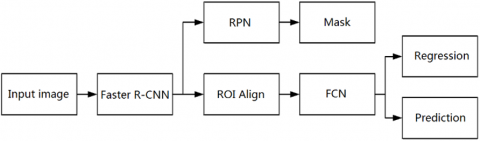Figure 1. The structure of Mask R-CNN

The Fast R-CNN (Figure 2) maps each candidate region to the feature layer of the CNN, and directly extracts the deep features from the region of interests (ROIs) on the feature layer, eliminating the need for constant input different regions of the image. Then, the extracted features were used to predict the ROI category on SoftMax, and create a bounding box regressor. The ROI-based extraction applies to images on various scales. Through end-to-end learning, the Fast R-CNN effectively improves the efficiency of the R-CNN.

## 2.png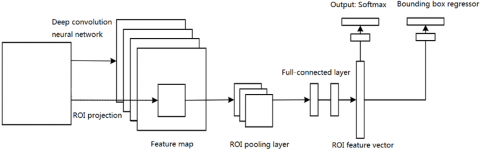Figure 2. The structure of Fast R-CNN

The Fast R-CNN has two parallel fully-connected output layers: the Softmax calculates the probability distribution of a single eigenvector in the k+1 category, while the bounding box regressor calculates the parameters of the bounding box. The two output layers are trained by a joint loss function:

$F\left(p, c, q^{c}, v\right)=F_{c l s}(p, c)+\delta[c \geq 1]_{g} F_{l o c}\left(q^{c}, \mathrm{v}\right)$     (1)

where, $\quad p=\left(p_{0}, \ldots, p_{k}\right) \quad ; \quad q^{c}=\left(q_{x}^{k}, q_{y}^{k}, q_{w}^{k}, q_{h}^{k}\right) \quad ; \quad v=v_{x}, v_{y}, v_{w}, v_{h} ; k$ is the number of categories; $F_{c l s}(p, c)=$ $-\log \left(p_{c}\right)$ is the logarithmic cost of real category $c ; F_{l o c}()$ is the loss due to regression.

The actual boundary $v$ and predicted bounding box $q^{c}$ of category $c$ can be calculated according to the definitions of the following parameters:

For $q^{c}=\left(q_{x}^{k}, q_{y}^{k}, q_{w}^{k}, q_{h}^{k}\right)$, each parameter can be defined as:

$\left\{\begin{array}{l}q_{x}=\frac{\left(o_{x}-c_{x}\right)}{c_{w}} \\ q_{y}=\frac{\left(o_{y}-c_{y}\right)}{c_{h}} \\ q_{w}=\log \left(\frac{o_{w}}{c_{w}}\right) \\ q_{h}=\log \left(\frac{o_{h}}{c_{h}}\right)\end{array}\right.$

where, $\left(O_{x}, O_{y}, O_{w}, O_{h}\right)$ are the center coordinates, border width and border height of real target, respectively; $\left(C_{x}, C_{y}, C_{w}, C_{h}\right)$ are the center coordinates, border width and border height of candidate area, respectively.

For the bounding regression layer, the loss can be defined as:

$F_{l o c}\left(q^{c}, \mathrm{v}\right)=\sum_{j \in(x, y, h, w)} \operatorname{Smooth}\left(q_{j}^{c}+v_{j}\right)$     (2)

where, Smooth $(y)=\left\{\begin{array}{lr}0.5 y^{2}, & |y|<1 \\ |y|-0.5, \text { otherwise }\end{array}\right.$.

After adding the mask branch, the loss function of each ROI can be computed by:

$F=F_{c l s}+F_{l o c}+F_{m a s k}$     (3)

For each ROI, the mask branch has an output of $K m * m$ dimensions, which includes $K$ masks of $m * m$ size; each mask involves $K$ categories.

Step 1. Input and preprocess the target image;

Step 2. Import the preprocessed image into a neural network for pre-training, yielding a feature map;

Step 3. Preset a ROI for each point in the feature map, producing multiple candidate ROIs;

Step 4. Send the candidate ROIs to the region proposal network (RPN) for binary classification to filter out some candidate ROIs;

Step 5. Perform the ROIAlign on the remaining ROIs;

Step 6. Classify and mask the ROIs.

4. Malicious Attack Detection Algorithm Based on Image Semantics

Despite its excellence in detecting image objects, the Mask R-CNN is not good at recognizing image attitude. As shown in Figure 3, our malicious attack detection algorithm is implemented by segmenting the semantics of webpage images, extracting the images containing human actions, recognizing the attitude in each image, judging the sensitive features, and integrating all features to identify malicious webpage.

## 3.png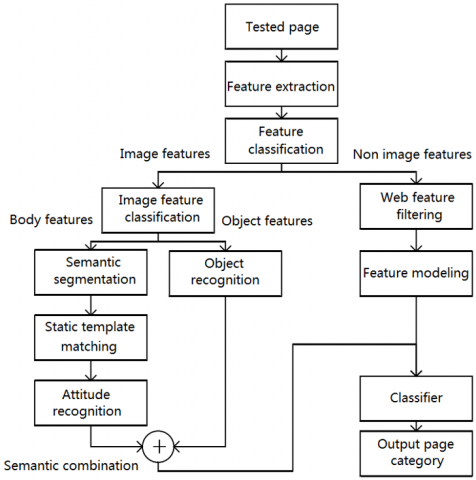Figure 3. The workflow of our algorithm

4.1 Kinect-based action matching

To ensure the accuracy of deep learning in attitude recognition, the target image was subjected to semantic segmentation, and the human body was extracted from the image. Then, the basic attitude of the human body was recognized based on the Kinect attitude database. After that, the semantics were combined based on environmental and human body features. Finally, the complex semantics of the image were predicted.

Because most images on malicious webpages are static, the malicious webpage detection method was improved through shape learning of training samples. To eliminate the influence of background noises and non-rigid deformation of human body, the semantically segmented image was sent to Kinect recognizer  to extract the sub-image that contains the attitude information. Then, the points of the human joints in the sub-image were matched with the Kinect attitude database, and the description eigenvectors of the corresponding attitudes were obtained.

The description thus obtained only expresses the meaning of the attitude. Except for some explicit pornographic actions, most of the basic actions are neutral semantics, which should be judged with the aid of the semantic information of other instances of semantic segmentation. For example, the recognized action “taking” can be combined with the instances “chips”, “dice” and “poker” into the semantic “gambling behavior”. Then, the target image can be determined as containing sensitive information.

Let $I$ be the attitude image of the corresponding area of mask in the input image, $\left\{S^{(I)}, M, S^{(N)}\right\}$ be a set of $N$ training samples in Kinect attitude database, $\left\{a^{(I)}, M, a^{(N)}\right\}$ be the semantic of the corresponding action, where $a^{(I)}$ is a word for action.

First, the distance between each sample in $I$ and $K$ was calculated as $D_{t}=D\left(I, K^{t}\right) .$ Then, the action semantic $a^{(\min )}$ corresponding to the sample $K^{(\text {min})}$ with the smallest value was selected as the semantic description of $I$ in $D_{t}$.

Let $\left(x^{(i)}, y^{(i)}\right)$ and $\left(x_{t}^{(i)}, y_{t}^{(i)}\right)$ be the coordinates of the $i$ -th joint point on $I$ and $K,$ out of the $M$ joint points. Then, the steps of Kinect-based action matching can be expressed as:

Step 1. Calculate the distance $d_{i}^{(t)}$ between the $i$ -th joint point on $I$ and $K:$

$d_{i}^{(t)}=\sqrt{\left(x^{(i)}-x_{t}^{(i)}\right)^{2}+\left(y^{(i)}-y_{t}^{(i)}\right)^{2}}$;

Step 2. Compute the total distance of all joint points on $I$ and $K:$

$D\left(\left(I, K^{t}\right)=\sum_{i=1}^{M} d_{i}^{(t)}\right.$;

Step 3. Calculate the Kinect sample index with minimum distance:

$\min =\arg \min _{k} D\left(\left(I, K^{t}\right)\right.$;

Step 4 . Take $a^{\text {min }}$ as the semantic description of $I$.

4.2 BPNN-based semantic inference

Most of the regions detected from a static image are basic neutral actions. Therefore, a BPNN was constructed based on feedback learning. For an image containing lots of suspected sensitive objects, the greater the degree of exposure of the human body, the larger the number of sensitive behaviors, and the more likely the image is sensitive. The BPNN algorithm can be implemented in the following steps:

Step 1. Initialize the number $k$ of basic actions of human body, the serial number $k_{i}$ of the $i$ -th attitude, and the number $N$ of sensitive objects.

Step 2. Calculate the length $d_{\text {touch}}$ of the shortest edge between the hand joint and the sensitive image:

$\vartheta_{\text {len}}=\left\{\begin{array}{ll}0, & d_{\text {touch}}=0 \\ \left(\frac{2 d_{\text {touch}}}{d_{\text {width}}+d_{\text {height}}}\right), & d_{\text {touch}}>0\end{array}\right.$;

where, $d_{\text {width}}$ and $d_{\text {height}}$ are the width and height of the image, respectively. Then, normalize $\vartheta_{\text {len}}$ to $\vartheta_{\text {touch}}:$

$\vartheta_{\text {touch}}=\left\{\begin{array}{lr}0, & \vartheta_{\text {len}}=0 \\ 1, & 1<\vartheta_{\text {len}} \leq 3 \\ 2, & 3<\vartheta_{\text {len}} \leq 7 \\ 3, & \vartheta_{\text {len}}>7\end{array}\right.$;

The sensitivity of the action is positively correlated with the closeness between the hand joint and the edge of the sensitive object, and peaks at the contact between the two objects.

Step 3. Compute the number of sensitive objects $n_{t h i n g}:$

$\vartheta_{\text {thing}}=\left\{\begin{array}{lr}0, & n_{\text {thing}}=0 \\ 1, & 1<n_{\text {thing}} \leq 3 \\ 2, & 3<n_{\text {thing}} \leq 5 \\ 3, & n_{\text {thing}}>5\end{array}\right.$;

If many suspected sensitive objects are detected in an image, the image is highly likely to contain sensitive semantics.

Step 4. Calculate the degree of exposure of human body:

$\vartheta_{\text {skin}}=\frac{s_{\text {skin}}}{s_{\text {body}}}$,

$\vartheta_{\text {naked}}=\left\{\begin{array}{lr}0, & \vartheta_{\text {skin}}=0 \\ 1, & 0<\vartheta_{\text {skin}} \leq 0.25 \\ 2, & 0.25<\vartheta_{\text {skin}} \leq 0.6 \\ 3, & \vartheta_{\text {skin}}>0.6\end{array}\right.$;

where, $S_{\text {skin}}$ is the degree of exposure; the greater the $S_{\text {skin}}$ value, the higher the possibility of sensitive action.

Step 5. Calculate the degree of sensitivity of the image:

$\vartheta_{i m a g e}=\left\{\begin{array}{lr}0, & n_{i m a g e}=0 \\ 1, & 1<n_{i m a g e} \leq 6 \\ 2, & 6<n_{i m a g e} \leq 12 \\ 3, & n_{i m a g e}>12\end{array}\right.$;

where, nimage is the number of sensitive images in the image set of a webpage. The greater the nimage value, the more likely to image is sensitive.

Step 6. Perform Bayesian probability combination of semantic and sensitive objects. Suppose there are $N$ types of sensitive semantics. Let $p\left(c_{j} | x\right)$ be the expected loss if sample $x$ is identified as $c$.

The classifier can be obtained according to Bayesian probability:

$h^{*}(x)=\arg \max _{c \in C} P(c | x)$,

$\vartheta_{\text {action}}=\left\{\begin{array}{lr}0, & 0<P(c | x) \leq 0.3 \\ 1, & 0.3<P(c | x) \leq 0.6 \\ 2, & 0.6<P(c | x) \leq 0.8 \\ 3, & P(c | x)>0.8\end{array}\right.$;

Then, a BPNN (Figure 4) with five input nodes can be established based on $\vartheta_{\text {touch}}, \vartheta_{\text {thing}}, \vartheta_{\text {naked}}, \vartheta_{\text {image}}$ and $\vartheta_{\text {action}},$ and used to compute the image semantic $a^{(I)}$ of target $y,$ creating a feature vector $f_{\text {action}}$ of malicious attitude transform.

## 4.png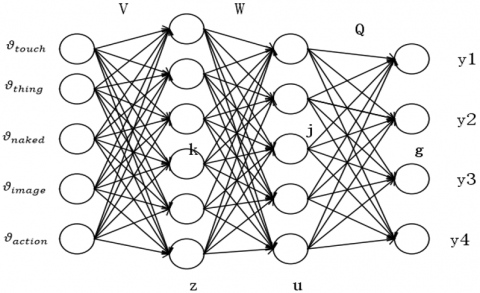Figure 4. The structure of the BPNN

4.3 Malicious webpage identification

The description of webpage feature function is shown in Table 1.

During malicious webpage detection, the page features, e.g. URL, keywords, page structure and registration information, are usually only described by part of the page information. Thus, the features cannot be generalized to different forms of webpages. These features should be considered comprehensively to improve the recognition accuracy of webpages with more text information.

Table 1. Webpage feature functions

 Functions Description $F_{1}$ The URL contains meaningless repeating letters. $F_{2}$ The number of links in the webpage is greater than $P_{1}$. $F_{3}$ The URL contains more unusual punctuations than $P_{2}$. $F_{4}$ The URL is longer than $P_{3}$ bytes. $F_{5}$ The URL takes the form of IP. $F_{6}$ The URL has a fake domain name. $F_{7}$ The URL contains sensitive words. $F_{8}$ The location of low-level domain name is abnormal. $F_{9}$ The webpage contains sensitive keywords. $F_{10}$ The webpage contains a fake certificate number. $F_{11}$ The URL contains the character @. $F_{12}$ The registration is less than $PF_{4}$ months. $F_{13}$ The webpage ranks below $FP_{5}$. $F_{14}$ The webpage is updated fewer than $P_{6}$ each month. $F_{15}$ The webpage has a fake document object model (DOM). $F_{16}$ The URL contains more paths than $P_{7}$.

For the text information contained in the target webpage, the functions $F_{1} \sim F_{16}$ were used to calculate each Boolean value in turn, and then a vector $f_{t e x t}$ was formed as the text feature of the webpage.

The semantic description feature of the image was obtained by the BPNN through joint point matching, and taken as the image keyword of the webpage. This feature was combined with the text feature $f_{t e x t}$ into the final feature $f=\left[f_{\text {text}}, f_{\text {action}}\right]$ to train the heuristic learner.

The classification and regression tree (CART) algorithm  were adopted to solve the detection of malicious webpage as binary classification problem. Suppose the classification problem contains $K=2$ categories. For a given sample set $D$ let $p_{k}$ be the probability that the sample points belong to the $k$ -th category. Then, the Gini value of the sample set can be calculated by:

$\operatorname{Gini}(D)=\sum_{k=1}^{K} \sum_{k^{\prime}=1, k \neq k^{\prime}}^{K} p_{k} p_{k^{\prime}}=1-\sum_{k=1}^{K} p_{k}^{2}$.

Next, the attribute with the minimum Gini value or regression variance of the child nodes was taken as the benchmark of node splitting, and the CART was constructed to classify the webpages.

5. Experiment Verification and Results Analysis

To verify its performance, our algorithm was subjected to malicious webpage recognition experiments, in comparison with the FCN and Mask R-CNN. The recognition performance was mainly measured by the prediction accuracy of the classifier for malicious webpages. The experimental parameters were configured as: $P_{1}=40, \quad P_{2}=5$ $P_{3}=80, P_{4}=3, P_{5}=1,000, P_{6}=10$ and $P_{7}=5$.

The COCO semantic dataset , which contains 91 common object categories, was selected as the training set of semantic segmentation. A total of 76,856 webpages published from January to December 2019 were randomly selected from the webpage security database PhishTank , and divided into a training set, a verification set and a test set at the ratio of 2:1:1.

Three independent experiments were carried out to evaluate the semantic segmentation, attitude recognition and webpage recognition of the proposed algorithm and the two contrastive algorithms on different datasets.

Table 2 lists the results of the three methods for semantic segmentation on COCO dataset. It can be seen that the proposed algorithm achieved comparable segmentation accuracy to that of Mask R-CNN with 19.2% less running time. This means our algorithm can reduce the computing load without sacrificing the accuracy.

Table 2. Comparison of image semantic performance

 Algorithm Average precision (AP) (%) Precision (%) Running time (s) FCN 83.6 82.7 4.43 Mask R-CNN 90.1 88.6 6.54 Proposed algorithm 90.2 88.5 5.29

Figure 5 provides an example of the recognition effects between the proposed algorithm and the Mask R-CNN.

## 5.png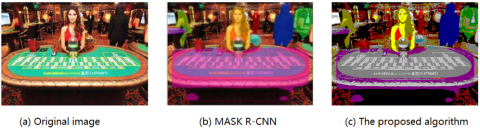Figure 5. An example of the recognition effects of different algorithms

Table 3 compares the results of the three methods for human attitude recognition.

Table 3. Comparison of attitude recognition performance

 FCN Mask R-CNN Proposed algorithm Attention 72.52% 87.2% 91.05% Bow 68.83% 73.72% 79.91% Raise hands 65.27% 72.96% 80.64% Walk 67.83% 77.25% 82.76% Handshake 64.89% 69.27% 75.92% Take 65.73% 80.15% 85.82% Lying 69.04% 79,51% 86.29% Side lying 68.33% 78.71% 85.49%

As shown in Table 3, the proposed algorithm was much more accurate than the Mask R-CNN in the recognition of human attitude.

Finally, the webpage data of PhishTank were divided into three subsets, according to the proportion of texts to images on the page: pages with more text (dataset A), pages with more images (dataset B), and test pages (dataset C). The three subsets add up to the whole dataset $D S_{\text {total}}$. Then, the proposed algorithm was compared with two malicious webpage detection algorithms on the dataset [17, 18].

It can be seen from Table 4 that the proposed algorithm was more accurate than the two contrastive algorithms, especially for pages containing lots of texts or images. This is because the contrastive algorithms rely on the DBN for webpage recognition. The DBN can realize self-supervised training, but perform poorly in discriminating pages containing images.

Overall, the proposed algorithm achieved ideal performance in semantic segmentation, attitude recognition and webpage classification. The high accuracy of malicious webpage detection is attributable to the synthetic use of semantic features and webpage features.

Table 4. Comparison of malicious webpage detection performance

 Algorithms Dataset A Dataset B Dataset C $\boldsymbol{D S}_{\text {total}}$ Algorithm 1  81.8% 73.5% 85.8% 79.7% Algorithm 2  83.8% 71.7% 83.9% 78.8% The proposed algorithm 85.7% 82.6% 87.6% 85.6%
6. Conclusions

Malicious webpages pose a serious threat to the information security of Internet users. However, the traditional methods for malicious webpage detection have not made full use of image information. This paper improves the Mask R-CNN to perform semantic segmentation of images, and derives attitude and other contextual semantics. The features of the target webpage were synthetized, and sent to the classifier for recognition. Each part of our algorithm was evaluated separated through experiments. The experimental results fully demonstrate the effectiveness of our algorithm.

Acknowledgement

This work was funded by the National Natural Science Foundation of China (Nos. 61862042, 61762062, 61601215, U1936120, U1636216); the National Key R&D Program of China (Nos. 2017YFB0802805, 2017YFB0801701); the Science and Technology Innovation Platform Project of Jiangxi Province (No. 20181BCD40005); the Fok Ying Tung Education Foundation of China (No. 171058); the Major Discipline Academic and Technical Leader Training Plan Project of Jiangxi Province (No. 20172BCB22030); the Primary Research & Development Plan of Jiangxi Province (Nos. 20192BBE50075, 20181ACE50033); the Jiangxi Province Natural Science Foundation of China (Nos. 20192BAB207019, 20192BAB207020); the Graduate Innovation Fund Project of Jiangxi Province (Nos. YC2019-S100, YC2019-S048); the Practice innovation training program of Jiangxi Province for college students (No. 20190403041).

References

 Shreeram, V., Suban, M., Shanthi, P., Manjula, K. (2010). Anti-phishing detection of phishing attacks using genetic algorithm. International Conference on Communication Control and Computing Technologies, Ramanathapuram, pp. 447-450. https://doi.org/10.1109/ICCCCT.2010.5670593

 Barraclough, P.A., Hossain, M.A., Tahir, M.A., Sexton, G., Aslam, N. (2013). Intelligent phishing detection and protection scheme for online transactions. Expert Systems with Applications, 40(11): 4697-4706. https://doi.org/10.1016/j.eswa.2013.02.009

 Hans, K., Ahuja, L., Muttoo, S.K. (2014). Approaches for web spam detection. International Journal of Computer Applications, 101(1): 38-44. http://dx.doi.org/10.5120/17655-8467

 Sur, C. (2019). DeepSeq: learning browsing log data based personalized security vulnerabilities and counter intelligent measures. Journal of Ambient Intelligence and Humanized Computing, 10(9): 3573-3602. https://doi.org/10.1007/s12652-018-1084-9

 Rao, R.S., Pais, A.R. (2019). Detection of phishing websites using an efficient feature-based machine learning framework. Neural Computing and Applications, 31(8): 3851-3873. https://doi.org/10.1007/s00521-017-3305-0

 Dérian, P., Héas, P., Herzet, C., Mémin, E. (2013). Wavelets and optical flow motion estimation. Numerical Mathematics: Theory, Methods and Applications, 6(1): 116-137. https://doi.org/10.1017/S1004897900001161

 Ramya, M., Krishnaveni, V., Sridharan, K.S. (2017). Certain investigation on iris image recognition using hybrid approach of Fourier transform and Bernstein polynomials. Pattern Recognition Letters, 94: 154-162. https://doi.org/10.1016/j.patrec.2017.04.009

 Li, C., Zhang, H.Y., Yang, X.P., Wang, F., Chen, Z.P. (2010). Dual range-based space target multi-attitude angles feature fusion recognition algorithm. Control and Decision, 25(9):1374-1378.

 Ognibene, D., Balkenius, C., Baldassarre, G. (2008). A reinforcement-learning model of top-down attention based on a potential-action map. The Challenge of Anticipation, 161-184. https://doi.org/10.1007/978-3-540-87702-8_8

 Gosavi, A. (2004). A reinforcement learning algorithm based on policy iteration for average reward: Empirical results with yield management and convergence analysis. Machine Learning, 55(1): 5-29. https://doi.org/10.1023/B:MACH.0000019802.64038.6c

 Zhao, D., Chen, Y., Lv, L. (2016). Deep reinforcement learning with visual attention for vehicle classification. IEEE Transactions on Cognitive and Developmental Systems, 9(4): 356-367. https://doi.org/10.1109/TCDS.2016.2614675

 Chiao, J.Y., Chen, K.Y., Liao, K.Y.K., Hsieh, P.H., Zhang, G., Huang, T.C. (2019). Detection and classification the breast tumors using mask R-CNN on sonograms. Medicine, 98(19): e15200. http://dx.doi.org/10.1097/MD.0000000000015200

 Zhang, Z. (2012). Microsoft Kinect sensor and its effect. IEEE Multimedia, 19(2): 4-10. https://doi.org/10.1109/MMUL.2012.24

 Gutiérrez-Esparza, J.C., Gómez-Hernández, J.J. (2017). Inverse Modeling Aided by the Classification and Regression Tree (CART) Algorithm. Geostatistics Valencia 2016, 805-819. https://doi.org/10.1007/978-3-319-46819-8_55

 Anderson, P., Fernando, B., Johnson, M., Gould, S. (2016). Spice: Semantic propositional image caption evaluation. European Conference on Computer Vision, 382-398. https://doi.org/10.1007/978-3-319-46454-1_24

 Geng, G.G., Lee, X.D., Zhang, Y.M. (2015). Combating phishing attacks via brand identity and authorization features. Security and Communication Networks, 8(6): 888-898. https://doi.org/10.1002/sec.1045

 Lin, C.F., Lin, S.F. (2013). Efficient face detection method with eye region judgment. EURASIP Journal on Image and Video Processing, 2013(1): 34. https://doi.org/10.1186/1687-5281-2013-34

 Iyengar, S., Hahn, K.S. (2009). Red media, blue media: Evidence of ideological selectivity in media use. Journal of Communication, 59(1): 19-39. http://dx.doi.org/10.1111/j.1460-2466.2008.01402.x